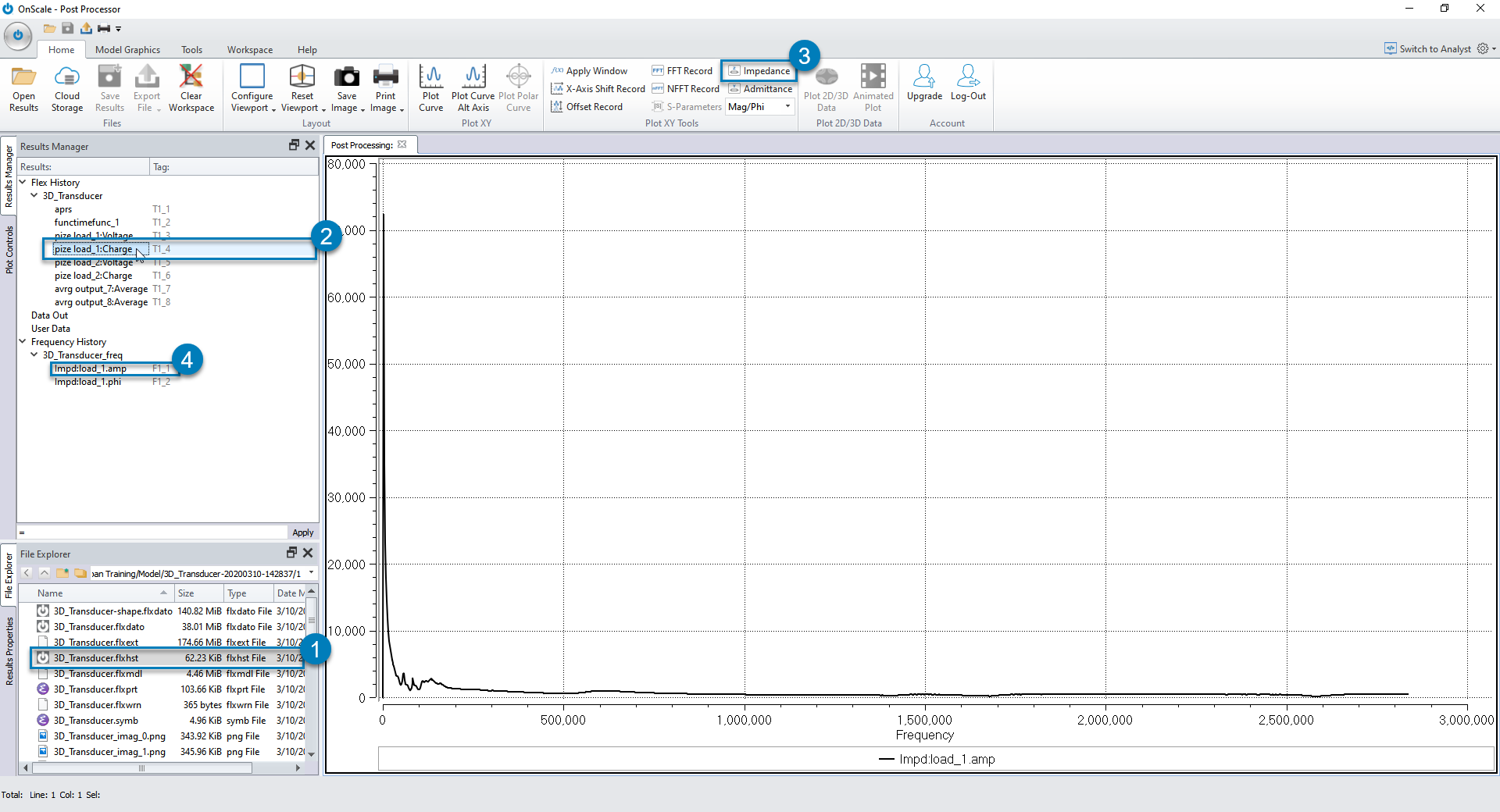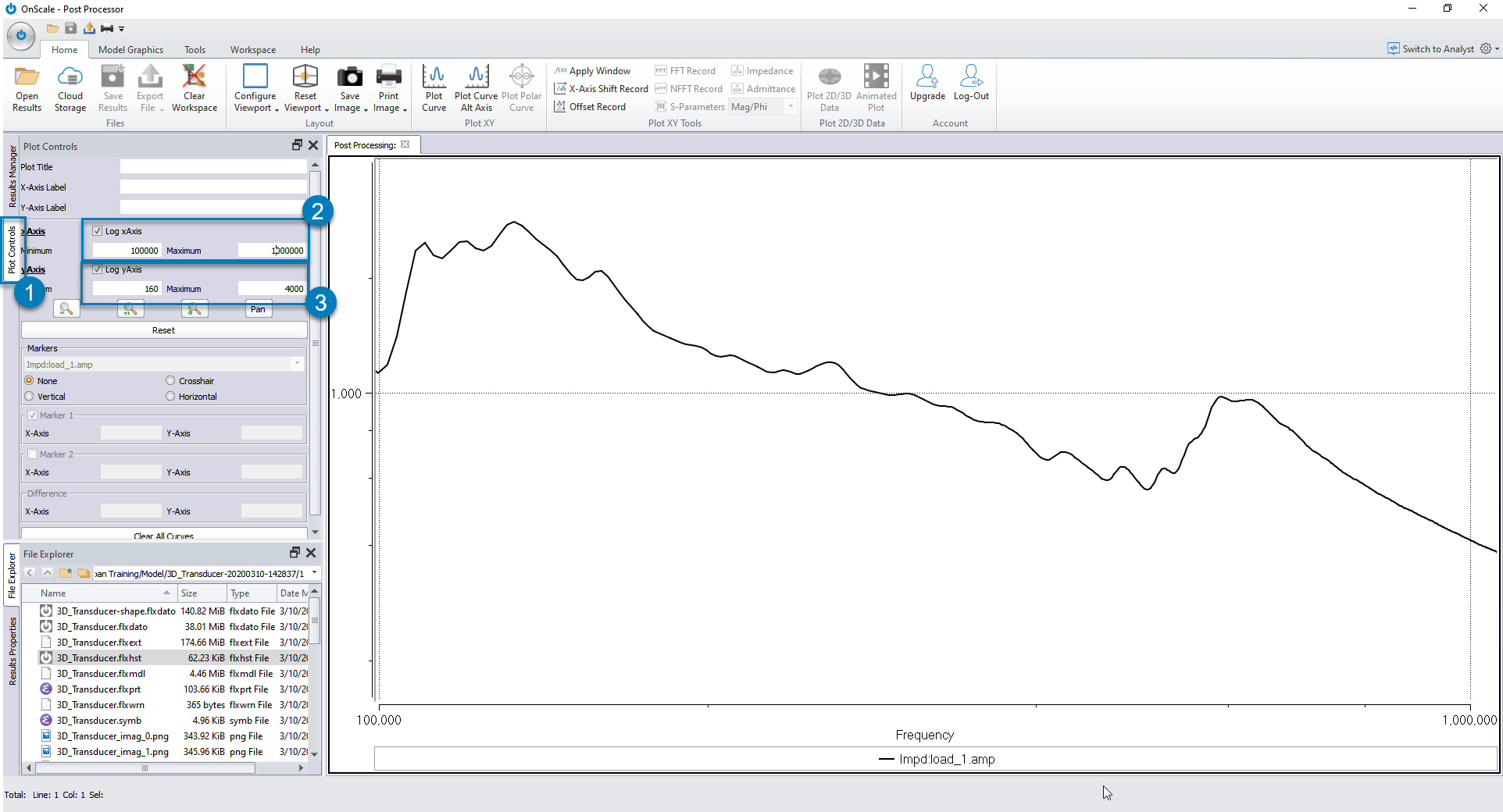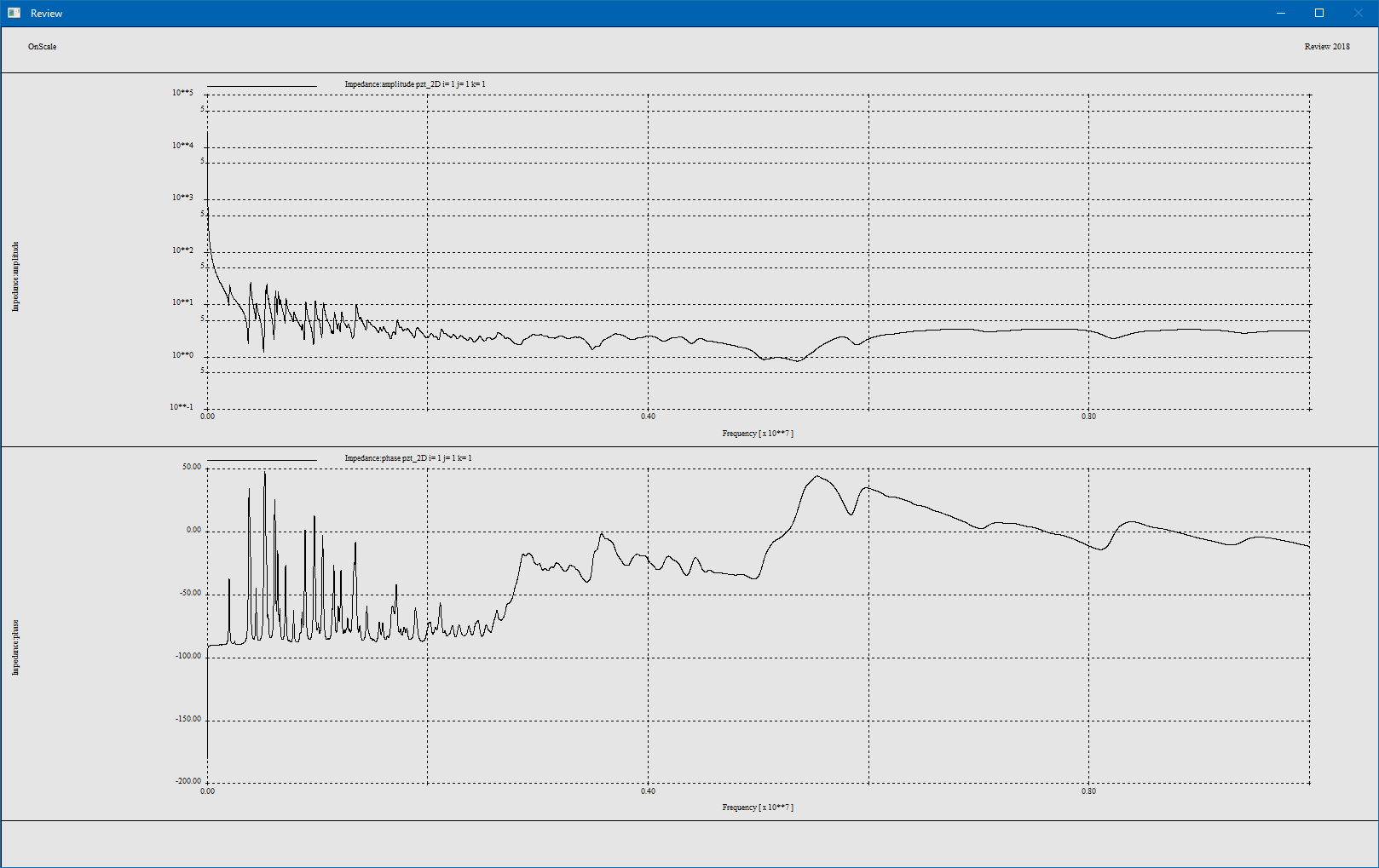# Calculating Impedance

The easiest way to calculate impedance in OnScale is by using the "Impedance" function available in the Post-process GUI. It is also possible to calculate the Impedance using Review Scripting Code.

## Calculating Impedance in OnScale Post-Process Module

1. Open you "XX.flxhst" data file which contains Time History Curve results from within OnScale Post-processor GUI
2. Click one time only on the "pize load_1:Charge" time history curve containing the discharge of the electrode 1
3. Click on the "Impedance" function which then becomes activated
4. Double click on the "Impd:load_1.amp" which then appears under Frequency History to display the amplitude of the Impedance curveNote: Click on the Image to display it in full screen

Only the portion close to the transducer device main frequency is important to us.

1. Click on the Plot Controls Onglet
2. Activate Log xAxis and enter minimum and maximum frequency values
3. Activate Log yAxis and enter minimum and maximum amplitude valuesNote: You can also zoom on the curve using the mouse using a mouse drag and drop.

Let's investigate now how to compute the impedance using review script code

## Calculating Impedance in Review with Script Code

Complex impedance is computed using time histories of voltage and charge therefore the following code must be included in the "XX.flxinp" input file to enable impedance to be extracted in Review:

`pout      histname electrode vq all      /* Volt/charge time histories 1 & 2      end`

Note: If you generated your input file with OnScale Designer Mode, this code should already be in your input file. Note that if you change "vq" into "vqi", you will also extract current at each electrodes.

Once the input file is run, it creates a history file that you can open by creating a "XX.revinp" Review script and paste the following code into it:

XX.revinp

`c Read the XX.flxhst file into a file f1text filename = InsertNameHere      /* Change filename as requiredread f1 '\$filename.flxhst'          /* Read time historyc Open a new file f2, then generate impedance curve using data from f1freq     file f2                             /* Local file to store results     type amp                            /* Results in Amplitude and Phase     impd f1 2 f1 3                      /* Calculate Impedance Magnitude     endc Plot the newly generate impedance curvegrph       type stnd                          /* Use standard graphics for Logarithmic plots       nvew 2       pset wndo 0 10.e6                  /* Set up X Scale       plot f2 1                          /* Plot Amplitude       set log y on        plot f2 2                           /* Plot Phase       endterm`

Note: Don't forget to change the "InsertNameHere" with the name XX of your time history flxhst file. You may need to change as well the "f1 2" and "f1 3" in the impd subcommand as voltage and current are not necessarily located in 2nd and 3rd position in your time history file. Finally, you need to set the frequency min and max values in the pset wndo command.

Your Review script must be located in the same directory as the '\$filename.flxhst' file

Let's now have a look at additional commands that you may need to add in your "freq" command in some special cases.

In some cases, the impedance plot is poorly discretized (or fractured). This is because the number of data points in the time history is insufficient to produce a suitably small frequency step size when the FFT was calculated.

The solution is to pad out the time history with extra zeros. Although this may sound like a cheat, it simply adds extra data points after the charge on the electrode in the model has already rung down to zero. This does not change the data; it merely extends the data set. The addition of the zero data points in the time set increases the number of points in the frequency set, thereby decreasing the frequency time step.

`freq     file f2                          /* Local file to store results     time pad 4                       /* Zero padding for better resolution     end    `

Another common problem with impedance plots is the addition of ringing resonances in the operational impedance, when the simulation has not run for long enough and the charge has not dissipated from the electrode. This truncation of the charge signal introduces spurious signals into the impedance characteristics. Windowing the new local file prevents generation of these frequencies.

`freq      file f2                          /* Local file to store results      wndo hann righ 0.15              /* Windowing to ensure signals terminate at zero      end`

## Calculation

The voltage and charge time history on an electrode where the drive function was applied are stored in records 2 and 3 which is what is used to calculate impedance.

`freq     file f2                             /* Local file to store results     type amp                            /* Results in Amplitude and Phase     impd f1 2 f1 3                      /* Calculate Impedance Magnitude     end`

By default, impedance data is output in magnitude and phase format; use of the type command can change this to real and imaginary. The impd command calculates the operational impedance based on arrays 2 and 3 in local file f1 and outputs the amplitude and phase to arrays 1 and 2, respectively, in local file f2.

## Plotting

Impedance curves can be plot using the grph command. The number of viewports is set using the nvew command and then plot is used to specify which curves to plot in the ports. Limits can be set on the X and Y axis using the pset command.

`grph       type stnd                          /* Use standard graphics for Logarithmic plots       nvew 2       pset wndo 0 10.e6                  /* Set up X Scale       plot f2 1                          /* Plot Amplitude       set log y on        plot f2 2                           /* Plot Phase       end`